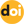Izvestiya vuzov. Yadernaya Energetika

The peer-reviewed scientific and technology journal. ISSN: 0204-3327

# Stochastic theory of zero power nuclear reactors. Part 2. Probability of degeneration for a branching process and some issues of estimating the probability of a nuclear accidenthttps://doi.org/10.26583/npe.2014.2.13

### UDC: 621.039.516

A formula has been derived to estimate the degeneration probability in which the conditional probability that the fission process will not stop by the time t is determined by using a system of non’linear differential equations presented in the paper. It follows from the formula that whatever the breeding ratio, a branching process with a probability of one will never arise in a nuclear reactor without an external excitation.

A general relationship has been found for an asymptotic value of probability that the fission process will stop in the nuclear reactor. It has been shown that Hansen’s model is a quadratic approximation of the general model and always overestimates this probability.

The paper presents a theoretical analysis which shows that Hansen’s model underestimates the probability of a nuclear accident as compared to the estimates derived by using the general model presented in the paper.

1. Volkov Yu. V. Stohasticheskaja teorija jadernyh reaktorov nulevoj moshhnosti. Chast’
2. Fizicheskaja i matematicheskaja modeli [Stochastic Theory of Zero Power Nuclear Reactors. Part 1. Physical and Mathematical Models]. Izvestiya vuzov. Yadernaya rnergetika. 2013, no.4, pp. 127-134.
3. Sevast’yanov B. A. Vetvjashhiesja processy [Branching Processes]. Мoscow, Nauka Publ., 1971. (in Russian)
4. Sudek G. Problemy kinetiki reaktora. V sb. Teorija jadernyh reaktorov [Aspects of Reactor Kinetics. In Reactor Theory] Мoscow, Gosatomizdat Publ., 1963. (in Russian)
5. Uhrig R. Statisticheskie metody v fizike jadernyh reaktorov [Statistical Methods in Reactor Physics]. Мoscow, Atomizdat Publ., 1974. (in Russian)
6. Gnedenko B. V., Kovalenko I. N. Vvedenie v teoriju massovogo obsluzhivanija [Introduction to Queueing Theory]. Мoscow, Nauka Publ., 1966. (in Russian)
7. Hansen G.E. Assembly of Fissionable Material in the Presence of a Weak Neutron Source. Nucl. Sci. Eng. 1960, v. 8, pp. 709-719.
8. Hankins D.E. Effect of Reactivity Addition Rate and of Weak Neutron Source on the Fission Yield of Uranium Solutions. Nucl. Sci. Eng. 1966, v. 26, pp. 110-116.
9. Shabalin E.P. Impul’snye reaktory na bystryh nejtronah [Fast Neutron Pulsed Reactors]. Мoscow, Atomizdat Publ., 1976. (in Russian)